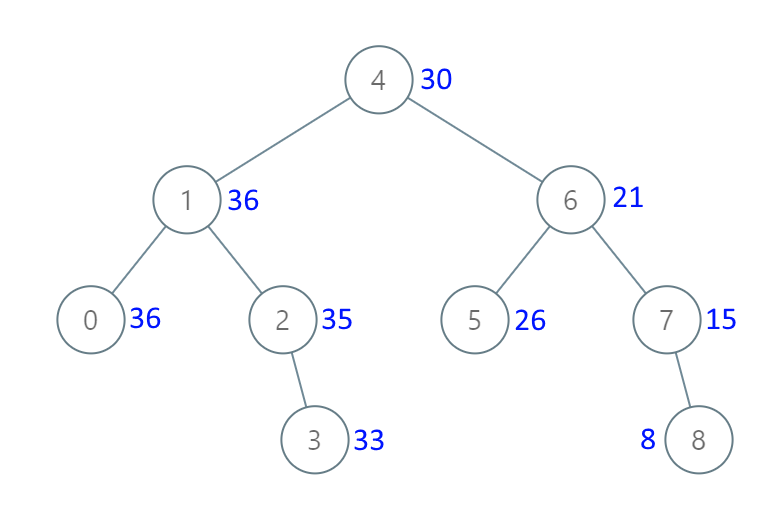# GeetCode Hub

Given the `root` of a Binary Search Tree (BST), convert it to a Greater Tree such that every key of the original BST is changed to the original key plus sum of all keys greater than the original key in BST.

As a reminder, a binary search tree is a tree that satisfies these constraints:

• The left subtree of a node contains only nodes with keys less than the node's key.
• The right subtree of a node contains only nodes with keys greater than the node's key.
• Both the left and right subtrees must also be binary search trees.

Note: This question is the same as 1038: https://leetcode.com/problems/binary-search-tree-to-greater-sum-tree/

Example 1:```Input: root = [4,1,6,0,2,5,7,null,null,null,3,null,null,null,8]
Output: [30,36,21,36,35,26,15,null,null,null,33,null,null,null,8]
```

Example 2:

```Input: root = [0,null,1]
Output: [1,null,1]
```

Example 3:

```Input: root = [1,0,2]
Output: [3,3,2]
```

Example 4:

```Input: root = [3,2,4,1]
Output: [7,9,4,10]
```

Constraints:

• The number of nodes in the tree is in the range `[0, 104]`.
• `-104 <= Node.val <= 104`
• All the values in the tree are unique.
• `root` is guaranteed to be a valid binary search tree.

/** * Definition for a binary tree node. * public class TreeNode { * int val; * TreeNode left; * TreeNode right; * TreeNode() {} * TreeNode(int val) { this.val = val; } * TreeNode(int val, TreeNode left, TreeNode right) { * this.val = val; * this.left = left; * this.right = right; * } * } */ class Solution { public TreeNode convertBST(TreeNode root) { } }